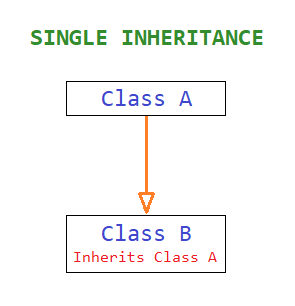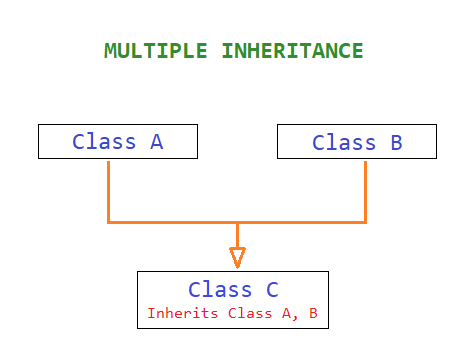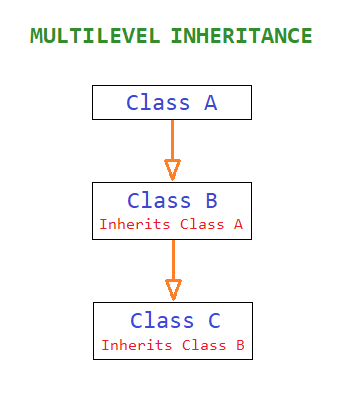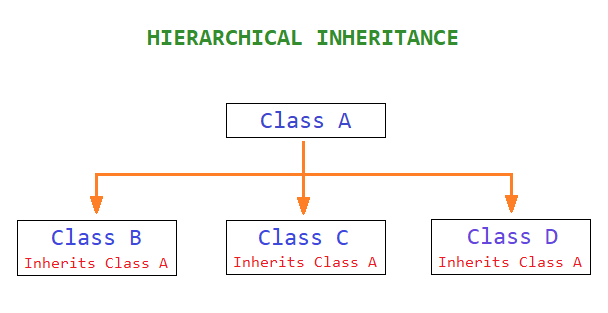# Types of Inheritance in Python Programming

###### OOPs in Python

In this lesson, we will understand how many types of Inheritance are their in Python and how to use them in class object programming.

## Types of Inheritance in Python

There are four types of inheritance available in python, and they are:

• Single Inheritance
• Multiple Inheritance
• Multilevel Inheritance
• Hierarchical Inheritance## Single InheritanceWhen a class inherits another class, this type of inheritance is called Single Inheritance. It is the most simple form of inheritance in python.

The above image shows an example of single inheritance in which class B inherits class A. Thus class A can be called a base class or parent class, and class B can be called a child class or subclass or derived class.

### Example of Single Inheritance

``````class ClassA:
def display(self):
print('In Class A')

class ClassB(ClassA):
def display(self):
ClassA.display(self)
print('In Class B')

x = ClassB()
x.display()``````

#### Output

```In Class A
In Class B```

## Multiple InheritanceWhen a class is derived by inheriting two or more classes, this type of inheritance is called Multiple Inheritance.

In the above image, class C has been derived by inheriting class A and class B.

### Example of Multiple Inheritance

``````class ClassA:
def display(self):
print('In Class A')

class ClassB:
def display(self):
print('In Class B')

class ClassC(ClassA, ClassB):
def display(self):
ClassA.display(self)
ClassB.display(self)
print('In Class C')

x = ClassC()
x.display()``````

#### Output

```In Class A
In Class B
In Class C```

## Multilevel InheritanceWhen a class is created by inheriting another derived class which is previously derived using another class, this type of inheritance is called Multilevel Inheritance.

In the above example, class C has been derived from class B, which is derived from class A.

### Example of Multilevel Inheritance

``````class ClassA:
def display(self):
print('In Class A')

class ClassB(ClassA):
def display(self):
ClassA.display(self)
print('In Class B')

class ClassC(ClassB):
def display(self):
ClassB.display(self)
print('In Class C')

x = ClassC()
x.display()``````

#### Output

```In Class A
In Class B
In Class C```

## Hierarchical InheritanceWhen two or more classes inherit the same base class, this type of inheritance is called Hierarchical Inheritance.

In the above image, classes B, C and D inherit the same base class A.

### Example of Hierarchical Inheritance

``````class ClassA:
def display(self):
print('In Class A')

class ClassB(ClassA):
def display(self):
ClassA.display(self)
print('In Class B')

class ClassC(ClassA):
def display(self):
ClassA.display(self)
print('In Class C')

class ClassD(ClassA):
def display(self):
ClassA.display(self)
print('In Class D')

x = ClassB()
y = ClassC()
z = ClassD()
x.display()
y.display()
z.display()``````

#### Output

```In Class A
In Class B
In Class A
In Class C
In Class A
In Class D```